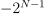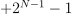/NumPy 1.17

# numpy.binary_repr

`numpy.binary_repr(num, width=None)` [source]

Return the binary representation of the input number as a string.

For negative numbers, if width is not given, a minus sign is added to the front. If width is given, the two’s complement of the number is returned, with respect to that width.

In a two’s-complement system negative numbers are represented by the two’s complement of the absolute value. This is the most common method of representing signed integers on computers . A N-bit two’s-complement system can represent every integer in the rangeto.

Parameters: `num : int` Only an integer decimal number can be used. `width : int, optional` The length of the returned string if `num` is positive, or the length of the two’s complement if `num` is negative, provided that `width` is at least a sufficient number of bits for `num` to be represented in the designated form. If the `width` value is insufficient, it will be ignored, and `num` will be returned in binary (`num` > 0) or two’s complement (`num` < 0) form with its width equal to the minimum number of bits needed to represent the number in the designated form. This behavior is deprecated and will later raise an error. Deprecated since version 1.12.0. `bin : str` Binary representation of `num` or two’s complement of `num`.

See also

`base_repr`
Return a string representation of a number in the given base system.
`bin`
Python’s built-in binary representation generator of an integer.

#### Notes

`binary_repr` is equivalent to using `base_repr` with base 2, but about 25x faster.

#### References

  Wikipedia, “Two’s complement”, https://en.wikipedia.org/wiki/Two’s_complement

#### Examples

```>>> np.binary_repr(3)
'11'
>>> np.binary_repr(-3)
'-11'
>>> np.binary_repr(3, width=4)
'0011'
```

The two’s complement is returned when the input number is negative and width is specified:

```>>> np.binary_repr(-3, width=3)
'101'
>>> np.binary_repr(-3, width=5)
'11101'
```

© 2005–2019 NumPy Developers
Licensed under the 3-clause BSD License.
https://docs.scipy.org/doc/numpy-1.17.0/reference/generated/numpy.binary_repr.html